﻿
Reach Us+44-1522-440391
Generation of Arbitrary Waveform Based on Optical Frequency Comb Using a Dual Parallel Mach-Zehnder Modulator and an Optical Filter

# Journal of Lasers, Optics & PhotonicsOpen Access

All submissions of the EM system will be redirected to Online Manuscript Submission System. Authors are requested to submit articles directly to Online Manuscript Submission System of respective journal.

# Generation of Arbitrary Waveform Based on Optical Frequency Comb Using a Dual Parallel Mach-Zehnder Modulator and an Optical Filter

Liu N and Wu S*
Key Laboratory of Specialty Fiber Optics and Optical Access Network, Shanghai University, China
*Corresponding Author: Wu S, Key Laboratory of Specialty Fiber Optics and Optical Access Network, Shanghai University, 99 Shangda Road, Shanghai 200444, China, Tel: +86-21-66137032, Email: [email protected]

Received Date: Mar 29, 2018 / Accepted Date: Apr 16, 2018 / Published Date: Apr 23, 2018

### Abstract

A scheme to generate optical arbitrary waveform based on a Dual Parallel Mach-Zehnder modulator (DPMZM) and an optical filter is proposed. One of the child MZMs (MZM-1) of the DPMZM is driven by the Ratio Frequency (RF) and DC bias voltage (Vbias-1), at the same time, the other child MZM (MZM-2) is driven by the RF multiplier and the DC bias voltage (Vbias-2). By adjusting the size of the RF driving voltages and the DC bias voltages, we can get a stable optical frequency comb with high side-comb suppression ratio (SCSR). Then optical frequency comb (OFC) matches with the tunable optical filter by adjusting the spectral line position and spacing, the waveforms of Gaussian, Trapezoid, square wave, sine, half-wave cosine and sinc-shaped pulse with a tunable repetition rate are generated.

Keywords: Dual Parallel Mach-Zehnder Modulator; Optical frequency comb; Arbitrary waveform; Optical filter; Optical communication systems

#### Introduction

With the development of optical information technology, the generation of photonic waveforms has great influence in many fields of science and engineering, such as optical communication , ultrawide band (UWB) communication , pulse radar  , microwave signal Generated  and so on. In recent years, how to produce optical arbitrary waveform with tunable repetition frequency and waveform is one of the focuses of current research. Optical arbitrary waveform generation technology is generally manipulated in the frequency domain pulse waveform corresponding to the spectral amplitude and phase parameters. The principle of the frequency domain method is to calculate the spectral components of the target time domain waveform by the Fourier transform theory, and then use the modulator to manipulate the amplitude and phase of the input signal spectrum according to the output signal spectrum to generate an arbitrary waveform in the time domain finally. In the Fourier synthesis approach , the first is to generate a stable, tunable spacing optical frequency comb from the input signal and then generate the desired waveform through an optical filter or pulse shaper [6,7]. A typical optical frequency comb is generated by a mode-locked laser, but the number of optical pulses is difficult to control, the pulse interval is indefinite, the repetition frequency is difficult to increase, and the price is more expensive . Generally, single/cascaded electro-optical modulators are relatively easy to produce optical frequency comb, mainly cascaded phase and intensity/phase modulation , the phase modulation after the cascaded intensity modulation has proved to be very important for the generation of optical frequency comb. However, in the above method, the power of the RF drive signal is very high, and the high power driver and phase shifter make the system complicated and unstable. Common electro-optic modulators include Mach-Zender Modulator (MZM), Phase Modulator (PM), and Polarization Modulator (PolM). Another solution to generate a flat and stable optical frequency comb is using a dual-drive Mach-Zehnder Modulator (DDMZM) [10-12]. Due to the good stability and high coherency of the resulting optical frequency comb, a 200fs (FWHM) ultrashort pulse can be produced using this scheme. Compared with the cascaded modulator, singlestage dual-drive can not only reduce component costs, but also simplify the system. Although waveforms can be optically generated by compensating the frequency chirp induced by the dual-drive Mach-Zehnder electro-optic modulation with dispersive fibers , the waveforms require zero dispersion for further propagation. However, these methods do not have very good advantages in producing common waveforms such as Gaussian, trapezoidal, triangular and rectangular. The photonics method for generating triangular waveforms has been verified by simulations using the DPMZM . The scheme uses a band-stop filter to filter out two unwanted carriers and adjusts the parameters of the modulator to obtain a full duty cycle triangle shape. Here, we propose a scheme based on DPMZM with RF multiplier to generate a stable optical frequency comb. Then OFC matches with the optical filter, various waveforms with repetition frequency of 10 GHz, 15 GHz and 20 GHz are generated, including Gaussian, Trapezoid, square wave, sine waveform, half-cosine and sinc-shaped pulse. In this scheme, there is no need for a cascaded modulator and high power driver, and RF signal applied to the modulator is much lower than the previous method.

The work structure of this article is as follows: In Section 1, review the research status of the optical frequency combs and the development of arbitrary waveform; In Section 2, we introduce the working principle and system structure of our scheme; In Section 3, the simulation results and analysis are presented; Finally, the content of this work is summarized in Section 4.

#### Principle and Structure

Figure 1 shows the system structure that generates an optical frequency comb and an arbitrary waveform. It consists of a continuous wave (CW) laser, a Polarization Controller (PC), a DPMZM, a frequency multiplier, an optical filter, an optical spectrum analyzer (OSA), and an optical sampling oscilloscope (OSO). The OSA is used to output the optical comb, and the OSO is used to view the time-domain waveform. The DPMZM is an integrated structure consisting of three child modulators with two parallel MZM-1 and MZM-2 embedded in the two arms of the main MZM-3 respectively. By changing the DC bias voltage on the main modulator MZM-3, the child modulator output light waves at the upper and lower arms produce a phase difference. MZM-1 is driven by the Ratio Frequency (RF) and DC bias voltage (Vbias-1). The MZM-2 is driven by the RF multiplier and the DC bias voltage (Vbias-2).

Figure 1: Schematic diagram of arbitrary waveforms generator (CW: continuous wave laser, PC: Polarization Controller, AMP: RF amplifier, OSA: optical spectrum analyzer, OSO: Optical Sample Oscilloscope).

The continuous wave laser is directed into the LiNbO3 DPMZM. At the first Y-branch of the DPMZM, it is split into two equal optical signals by power. After Mach-Zehnder modulator, in the second Y-branch waveguide interfere with each other to form a flat and stable optical frequency comb.

Assume that the optical signal input to the DPMZM is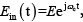, EMZM-1 and EMZM-2 represent the DPMZM output signals of the upper and lower arms. EMZM-1 − and EMZM-2 can be written as :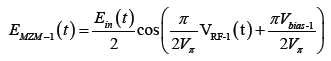(1)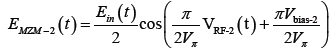(2)

Where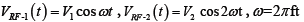represent the angular frequency of the RF driving signal, f represent the frequency of the RF driving signal, V1 and V2 are the RF signal amplitudes of MZM-1 and MZM-2, Vbias-1, Vbias-2 and Vbias-3 are the DC bias voltage of MZM-1, MZM-2 and MZM-3 respectively, Vπ is the half-wave voltage of the child MZMs. After the optical signal at the output of DPMZM can be written as: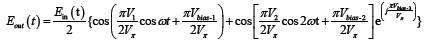(3)

According to the Bessel function, Eout(t ) can be written as: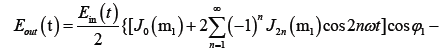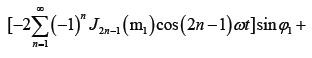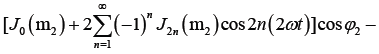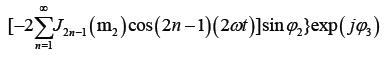(4)

Where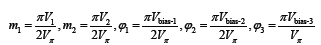and Jn(.) indicates the nth-order Bessel function. Optical carrier harmonic, 1storder, 2nd-order, 3rd-order and 4th-order sidebands can be written as: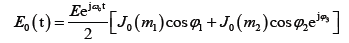(5)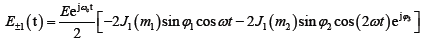(6)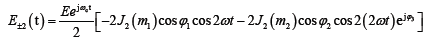(7)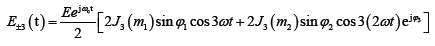(8)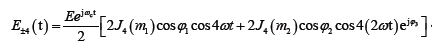(9)

From the Bessel plot as shown in Figure 2, it can be seen that there are multiple independent variables corresponding to the same Bessel function value, so the parameter for obtaining the same quality optical comb is not unique. It can be seen from the above equation that the intensity of the output optical frequency comb can be controlled by controlling the RF driving voltage V1 and V2. By adjusting the size of the RF driving voltage V1, V2 and the DC bias voltage Vbias-1, Vbias-2, Vbias-3, the intensity of each optical frequency comb can be controlled.

Figure 2: Relationship between the magnitude of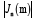and the modulation index m.

Compared with the other principle of generating the optical frequency comb, the frequency-doubled DPMZM requires less RF drive voltage, a high side-comb suppression ratio (SCSR) and more stable optical frequency comb can be obtained. By adjusting the five variables of V1, V2, Vbias-1, Vbias-2 and Vbias-3, we can get more optical frequency comb with a better flatness.

For arbitrary periodic function f(t) of period T, its Fourier series expansion can be written as :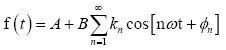(10)

Where A and B represent constants,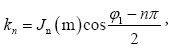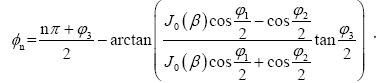According to eqn. (10), we take the generation periodic square wave and half-wave cosine as an example and it can be written as: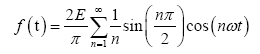(11)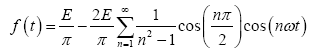(12)

By adjusting the spectral line position and spacing of OFC, we need select a set of interest lines from OFC through tunable optical bandpass filter and square wave and half-wave are generated. When special optical filters are implemented, various waveforms can be generated.

#### Simulation Results and Analysis

We carry out a simulation by VPI transmission Maker to verify the performance of our proposed scheme. A continuous wave laser is launched from laser source at a frequency of 193.1 THz and the line width is 10 MHz. The output power of continuous wave laser is 1 mW. The half-wave voltage of the two child MZMs is 3.5V and they are with a same extinction ratio of 35 dB. The frequency of the first RF is set to 5 GHz, and the frequency of the second RF is 10 GHz by a frequency multiplier. A tunable optical band-pass filter followed after the DPMZM, when the OFC matches with the optical filter, one group of spectral lines of interest is filtered. The center wavelength and the bandwidth of the optical band-pass filter are tunable. The optical bandpass filter has steep edge.

OFC of different amplitude and phase are generated by adjusting the five variables of V1, V2, Vbias-1, Vbias-2 and Vbias-3. When the amplitudes of RF signals and DC bias voltages set V1=0.027, V2=0.013, Vbias-1=0, Vbias-2 =1.1 and Vbias-3=3.5, a high SCSR and more stable optical frequency comb are generated. By selecting two spectral lines from OFC through suitable optical band-pass filter, a repetition rate of 10 GHz sine wave is generated as shown in Figure 3b. By adjusting the driving frequency, a repetition rate of 20GHz sine wave is generated as shown in Figure 3d.

Figure 3: Simulation results: (a)(c) are selected two spectral lines spaced by 10GHz, 20GHz respectively; (b)(d) are corresponding sine wave of (a)(c) respectively; the period of(b)(d)are 100ps and 50ps respectively.

In this scheme, we also propose and simulate the generation of sincshaped pulse sequence with a high SCSR and ultra-flat optical frequency comb based on a single DPMZM. By adjusting the five variables of V1, V2, Vbias-1, Vbias-2 and Vbias-3, five comb lines with a spacing of 10 GHz can be generated, as shown in Figure 4a. The flatness is within 0.5 dB and the SCSR is 24.5dB. In the time domain, the sinc-shaped pulses with a repetition rate of 10GHz are generated as shown in Figure 4b. The combination of optical frequency comb and sinc-shaped pulse sequence will play a greater role in optical communication system .

Figure 4: Simulation results: (a) is selected five spectral lines spaced by 10 GHz; (b) is the corresponding sinc-shaped pulse, the period of (b) is 100ps.

When special optical filters are implemented, various waveforms can be easily generated. In Figure 5, the simulation results of Gaussian, trapezoid, square wave and half-wave cosine with a repetition rate of 10GHz are generated. In our scheme, a 15 GHz repetition rate also can be obtained. The simulation results are shown in Figure 6. It means that different repetition rate can be generated with the same components by adjusting the driving frequency.

Figure 5: Simulation results: (a)(c)(e)(g) are selected spectral lines spaced by 10GHz; (b)(d)(f)(h) are the corresponding waveforms of (a)(c)(e)(g) respectively; the period of (b)(d)(f)(h) are 100ps.

Figure 6: Simulation results: (a) Square wave, (b) Half-wave cosine, (c) Gaussian wave, (d) Trapezoidal wave (waveforms generation with the repetition rates of 15 GHz.

#### Conclusion

We propose and demonstrate a scheme to generate optical arbitrary waveforms based on optical frequency comb generated with a DPMZM and a tunable optical filter. Compared with other scheme of generating optical frequency comb, our scheme requires less RF drive voltage and high side-comb suppression ratio (SCSR) and more stable optical frequency comb can be obtained by adjusting five variables. When the interval of optical frequency comb matches with the optical filter, the waveform of Gaussian, Trapezoid, square wave, sine, half-wave cosine and sinc-shaped pulse with a tunable repetition rate are generated. The tunable repetition and less RF drive voltage might be very attractive for arbitrary waveform generation.

#### References

Citation: Liu N, Wu S (2018) Generation of Arbitrary Waveform Based on Optical Frequency Comb Using a Dual Parallel Mach-Zehnder Modulator and an Optical Filter. J Laser Opt Photonics 5: 180. DOI: 10.4172/2469-410X.1000180

Copyright: © 2018 Liu N, et al. This is an open-access article distributed under the terms of the Creative Commons Attribution License, which permits unrestricted use, distribution, and reproduction in any medium, provided the original author and source are credited.

Select your language of interest to view the total content in your interested language

##### Recommended Journals
Viewmore
###### Article Usage
• Total views: 5366
• [From(publication date): 0-2018 - Sep 22, 2019]
• Breakdown by view type
• HTML page views: 5253

## Post your commentCan't read the image? click here to refresh
###### Peer Reviewed Journals

Make the best use of Scientific Research and information from our 700 + peer reviewed, Open Access Journals

International Conferences 2019-20

Meet Inspiring Speakers and Experts at our 3000+ Global Annual Meetings

Top